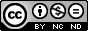## Friday, February 17, 2012

### How to plot a cross shaped axis when using gnuplot

It is always asked by my friends that how to plot a graph with cross shaped axis when using gnuplot. To the best of my knowledge, there is not such a built in axis style in gnuplot. So you have to draw the axis manually. Here a script is provided. You can just use it. If you would like to make some improvements, It is welcomed.

```#frame.gnuplot
#Author: 数声风笛离亭晚，我想潇湘君想秦
##########################################################
#Utility: plot a "+" style axis

#Usage:
#call "frame.gnuplot" "ox" "oy" "xmin" "xmax" "ymin"\
#     "ymax" "dx" "dy"

#Parameters:
#(Frame_ox,Frame_oy):     The position of the origin
#(Frame_xmin,Frame_ymin): The bottum-left point
#(Frame_xmax,Frame_ymax): The top-right point
#Frame_dx:                The interval of x-ticks
#Frame_dy:                The interval of y-ticks

#Before calling this script, Frame_xtic_len and
#Frame_ytic_len can be set to control the length
#of the corresponding tick length. The length is
#measured in graph coordinate. The default values
#are 0.02 and 0.01.

#Example:
#Frame_xtic_len = 0.01
#Frame_ytic_len = 0.01
#call "frame.gnuplot" "0." "1.0" "-10." "10." "0." \
#     "2." "2." "0.2"
#plot sin(x)+1.
##########################################################
Frame_ox=\$0
Frame_oy=\$1
Frame_xmin=\$2
Frame_xmax=\$3
Frame_ymin=\$4
Frame_ymax=\$5
Frame_dx=\$6
Frame_dy=\$7

#set the default value of Frame_x(y)tic_len
if (!exist("Frame_xtic_len")) Frame_xtic_len=0.02
if (!exist("Frame_ytic_len")) Frame_ytic_len=0.01
#no plot of the default border and tics
set border 0
unset tics
#set the xrange and yrange to the user defined value
set xrange [Frame_xmin:Frame_xmax]
set yrange [Frame_ymin:Frame_ymax]
#draw the axis
set arrow from graph 0, first Frame_oy to graph 1.03,\
first Frame_oy size graph 0.02,15,60 fill ls -1
set arrow from first Frame_ox, graph 0 to first Frame_ox,\
graph 1.03 size graph 0.015,15,60 fill ls -1
#draw the ticks
set for [i=0:int((Frame_xmax-Frame_xmin)/Frame_dx)] \
arrow from Frame_xmin+i*Frame_dx,Frame_oy to \
Frame_xmin+i*Frame_dx,Frame_oy+ \
set for [i=0:int((Frame_ymax-Frame_ymin)/Frame_dy)] \
arrow from Frame_ox,Frame_ymin+i*Frame_dy to \
Frame_ox+(Frame_xmax - Frame_xmin)*Frame_ytic_len, \
#draw the labels with the ticks
set for [i=0:int((Frame_xmax-Frame_xmin)/Frame_dx)] \
label sprintf("%3.1f",Frame_xmin+i*Frame_dx) at \
Frame_xmin+i*Frame_dx,Frame_oy center offset 0,-0.75
set for [i=0:int((Frame_ymax-Frame_ymin)/Frame_dy)] \
label sprintf("%3.1f",Frame_ymin+i*Frame_dy) at \
Frame_ox,Frame_ymin+i*Frame_dy right offset -0.75,0
```

Now let us show how this script is used by an example.

```Frame_xtic_len = 0.01
Frame_ytic_len = 0.01
call "frame.gnuplot" "0." "1.0" "-10." "10." "0." \
"2." "2." "0.2"
plot sin(x)+1. notitle
```Fig.1 Cross shaped axis plotted by gnuplot